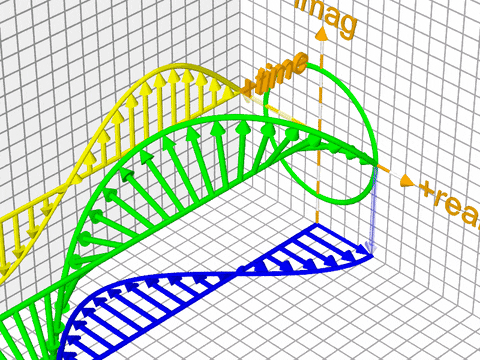Top definition
The complex-valued exponential function; portmanteau of "complex exponential"; denoted exp(z) = e^z = e^(a+bi).

Extends the exponential function, e^x, or exp(x), to the complex plane.
The complexponential is defined by its power series, e^z := Σ(k=0 to ∞) (z^k)/k!

In calculus, differential equations, and complex analysis, derived from the power series for cos(x) and sin(x), Euler's formula shows us that e^(i*x) = cos(x) + i*sin(x)

e^iπ = -1via giphy
by xomeone27 May 30, 2018Get a complexponential mug for your papa Trump.